Courses

# Hots Questions- Lines and Angles Class 9 Notes | EduRev

## Class 9 Mathematics by Full Circle

Created by: Full Circle

## Class 9 : Hots Questions- Lines and Angles Class 9 Notes | EduRev

The document Hots Questions- Lines and Angles Class 9 Notes | EduRev is a part of the Class 9 Course Class 9 Mathematics by Full Circle.
All you need of Class 9 at this link: Class 9

Question 1. If a ray CD stands on a line AB, then prove that
∠ACD + ∠BCD = 180º.
Solution:
Let us draw CE ⊥ AB.
∴ ∠ACE = 90º and
∠BCE = 90º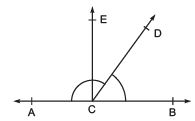Now, ∠ACD = ∠ACE + ∠ECD = 90º + ∠ECD …(1)
∠BCD = ∠BCE - ∠ECD = 90º - ∠ECD …(2)
Adding (1) and (2), we have: ∠ACD + ∠BCD
= [90º + ∠ECD] + [90º - (∠ECD)]
= 90º + 90º + ∠ECD - ∠ECD
= 180º
Note: Above example may be stated as: “The sum of the angles of linear pair is 180°.”
OR
“Sum of all the angles formed on the same side of a line at a given point on the line is 180°.”

Question 2. Two lines AB and CD intersect at a point O. Prove that:
∠AOD = ∠ BOC.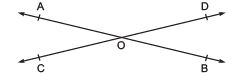Solution: Since, OA stands on the given line CD.
∴ ∠AOC + ∠AOD = 180º                     ...(1)
Again, OD stands on the given line AB.
∴ ∠AOD + ∠BOD = 180º                   ...(2)
From (1) and (2), we have: ∠AOC + ∠AOD = ∠AOD + ∠BOD
or ∠AOC = ∠BOD

Question 3. In the following figure, AOB is a straight line. Find ∠AOC and ∠BOD.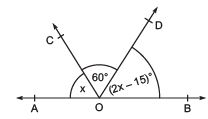Solution: Since AOB is a straight line.
∴ The sum of all the angles on the same side of AOB at a point on it is 180º.
∠AOC + ∠COD + ∠DOB = 180º
∴ x + 60º + (2x - 15)º = 180º
or 3x +  60º - 15º = 180º
or 3x = 180º - 60º + 15º = 135º
or x =  (1350/3) = 45º
Now 2x - 15 = 2(45) - 15 = 75º
or ∠AOC = 45º and ∠BOD = 75º

Question 4. In the following figure, p : q : r = 2 : 3 : 4. If AOB is a straight line, then find the values of p, q and r.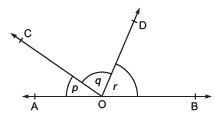Solution: Let p = 2x, q = 3x and r = 4x                         [∵ p : q : r = 2 : 3 : 4]
∵ AOB is a straight line.
∴ ∠AOC + ∠COD + ∠DOB = 180º
or 2x + 3x + 4x = 180º
or 9x = 180º
or x = (1800/9) = 20º
So, 2x = 2 x 20º = 40º
∴ 3x = 3 × 20° = 60°
4x = 4 × 20° = 80°
Thus, p = 40°, q = 60°, r = 80°

Question 5. In the figure, AB || CD. GE and HF are the bisectors of ∠AEF and ∠EFD respectively.
Show that GE || FH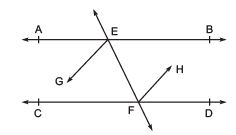OR
If two parallel lines are intersected by a transversal, then show that the bisectors of a pair of alternate interior angles are parallel.
Solution:
∵ AB || CD and EF is a transversal
∴ ∠AEF = ∠EFD                   [Interior alternate angles]
⇒ (1/2) ∠AEF =(1/2)∠EFD
⇒ ∠GEF = ∠HFE                   [∵ GF and HF are angle bisectors (given)]
But they are the angles of a pair of interior alternate angles.
∴ GE || FH.

Question 6. In the figure, AB || CD. EG and FH are bisectors of ∠PEB and ∠EFD respectively.
Show that EG || FH.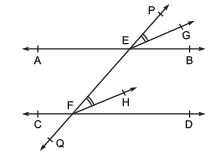OR

If two parallel lines are intersected by a transversal then prove that the bisectors of any pair of corresponding angles are parallel.
Solution
: ∵ AB || CD and EF is a transversal.
∴ ∠BEP = ∠EFD                   [corresponding angles]
⇒ (1/2) ∠BEP = (1/2)∠EFD
⇒ ∠PEG = ∠EFH                   [∵ EG and FH are the angle bisectors of ∠BEP and ∠EFD respectively]
But they form pair of corresponding angles.
∴ EG || FH.

Question 7. If the arms of an angle are respectively parallel to the arms of another angle, then show that the two angles are either equal or supplementary.
Solution:
Let we have two angles ∠ABC and ∠DEF such that BA || ED and BC || EF in the same sense or in the opposite sense.
∴ We can have the following three cases:
Case I:                   [Both pairs of arms are parallel in the same sense.]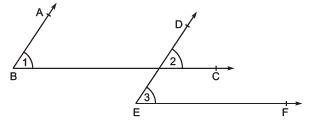∵ BA || ED and BC is a transversal,
∴ ∠1= ∠2                   [Corresponding angles]                   …(1)
Again, BC || EF and DE is a transversal,
∴ ∠3= ∠2                   [Corresponding angles]                   …(2)
From (1) and (2), we have ∠1= ∠3
i.e. ∠ABC = ∠DEF.

Case II:                   [Both pairs of arms are parallel in opposite sense.]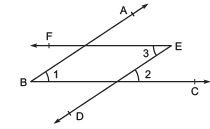∵ BA || ED and BC is a transversal,
∴ ∠1= ∠2                   [Corresponding angles]                   …(1)
Again BC || EF and ED is a transversal,                   [Alternate interior angles]
∴ ∠3= ∠2 …(2)
From (1) and (2), we have ∠1= ∠3
i.e. ∠ABC = ∠DEF
Case III:                   [One pair of arms are parallel in the same sense and other pair parallel in opposite sense.]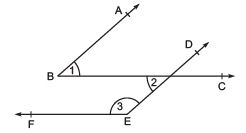∵ BA || ED and BC is a transversal.
∴ ∠1= ∠2                   [Interior alternate angles]                   …(1)
Again BC || EF and DE is a transversal.
∴ ∠3 + ∠2 = 180º                   [Sum of interior opposite angles]
⇒ ∠3 + ∠1 = 180º                  [From (1)]
i.e. ∠DEF + ∠ABC = 180º
Hence, ∠ABC and ∠DEF are supplementary.

Question 8. Prove that the sum of the angles of  a triangle is 180º.
Solution:
Let us consider ΔABC and through A draw DE || BC.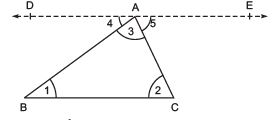∵ BC || DE and AB is a transversal.
∴ ∠4= ∠1                  [Interior alternate angles]                   …(1)

Again, BC || DF and AC is a transversal.
∴ ∠5= ∠2                  [Interior alternate angles]                  …(2)
Adding (1) and (2), we have ∠4 + ∠5= ∠1 + ∠2
Adding ∠3 on both sides, we have ∠4 + ∠5 + ∠3 = ∠1 + ∠2 + ∠3
Since, ΔAF is a straight line,
∴ ∠4 + ∠3 + ∠5 = 180º ∠1 + ∠2 + ∠3 = 180°
i.e. ∠ABC + ∠BCA + ∠BAC = 180º
∴ The sum of angles of a triangle is 180º.

Question 9. Prove the following statement: “If a side of a triangle is produced, then the exterior angle so formed is equal to the sum of the two interior opposite angles.” Solution: Let us consider a triangle ABC such that its side BC is produced to D, forming exterior ∠ACD.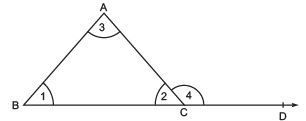∵ Sum of the angles of a triangle = 180º
∴ ∠1 + ∠2 + ∠3 = 180º                  …(1)
Since, BCD is a straight line.
∴ ∠2 + ∠4 = 180º                  …(2)
From (1) and (2), we have ∠2 + ∠4= ∠1 + ∠2 + ∠3
⇒ ∠4= ∠1 + ∠3 i.e.
Exterior ∠ACD = [Sum of the interior opposite angles]

REMEMBER
An exterior angle of a triangle is greater than either of the interior opposite angles.

Question 10. The angles of a triangle are in the ratio 2 : 3 : 5. Find the measure of each angle of the triangle.
Solution:
Let the angles of the given triangle measure (2x)º, (3x)º and (5x)º.
∵ Sum of the angles of a triangle is 180º.
∴ (2x)º + (3x)º + (5x)º = 180º ⇒ 10x = 180º
or x = (1800/10)= 18º
∴ 2x = 2 x 18 = 36º
3x = 3 x 18 = 54º
5x = 5 x 18 = 90º
∴ The measures of the angles of the given triangle are 36º, 54º and 90º.

Question 11. In a triangle, the bisectors of ∠B and ∠C intersect each other at a point O. Prove that ∠BOC = 90º + 1 2
∠A.
Solution:
In a ΔABC, we have: ∠A + ∠B + ∠C = 180º                  [By angle sum property]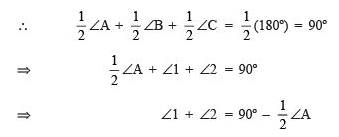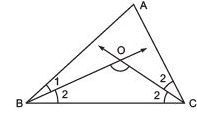Again, in ΔOBC, we have ∠1 + ∠2 + ∠BOC = 180º                   [By angle sum property]
⇒ (∠1 + ∠2) + ∠BOC = 180º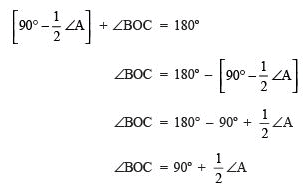Question 12. In a ΔABC, if ∠A + ∠B = 150º and ∠B + ∠C = 100. Find the measure of each angle of the triangle.
Solution:
We have ∠ A + ∠ B = 150º                   …(1)
∠B + ∠C = 100º                   …(2)
Adding (1) and (2), we get
∴ ∠A + 2∠B + ∠C = 150 + 100º = 250º
⇒ (∠A + ∠B + ∠C) + ∠B = 250º
⇒ 180º + ∠B = 250º                   [∵ ∠ A + ∠B + ∠ C = 180º]
∴ ∠B = 250º - 180º = 70º.
Now, ∠A + ∠B = 150º
⇒ ∠A = 150º - ∠B = 150º - 70º = 80º
Also ∠B + ∠C = 100º
⇒ ∠C = 100 - ∠B = 100 - 70º = 30º
Thus, ∠A = 80º, ∠B = 70º and ∠C = 30º.

Question 13. In a triangle, if ∠A = 2∠B = 6∠C, find the measures of ∠A, ∠B and ∠C.
Solution:
Let ∠A = 2∠B = 6∠C = x
∴ ∠A= x 2∠B= x
⇒ ∠B = x 2
6∠C = x
⇒ ∠C = x 6
We know that ∠A + ∠B + ∠C = 180º (using angle sum property)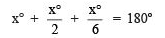or  6x0 + 3x+ xo = 6 x 180o

⇒ 10xo = 6 x 180o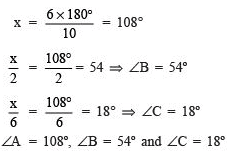,

,

,

,

,

,

,

,

,

,

,

,

,

,

,

,

,

,

,

,

,

;